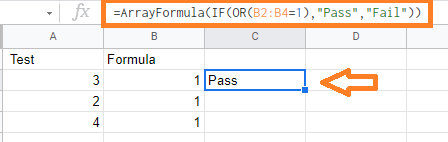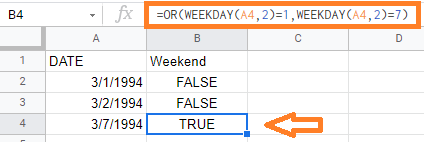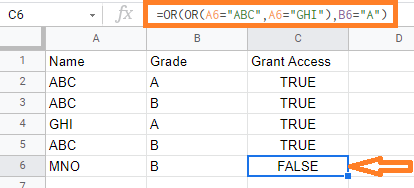# How to Use OR Function in Google Sheets With Examples (Logical Functions)

Google Sheets OR function returns TRUE if any of the provided arguments are logically true and FALSE if all the provided arguments are logically false. This function is useful when you want to evaluate a set of conditions in Google Sheets.

Also, we can combine the Google Sheets OR function with other functions to perform various mathematical operations. However, if the specified range contains no logical values, the google sheets OR function returns #VALUE! error. Let’s learn everything about OR Function with the help of Google Sheet tips provided on this page.

## OR Function Syntax in Google Sheets

=OR(logical_expression1,[logical_expression2,…])

• logical_expression1: A cell that contains an expression that represents a certain logical value, such as TRUE or FALSE. This will be the first condition that you want to validate.
• logical_expression2: This is optional. Additionally, reference to cells containing expressions representing logical values, such as TRUE or FALSE can be used.

## OR Function in Google Sheets With Other Functions

OR function in Google Sheets can be used with other formulas to be more efficient. For example, we have two conditions namely the IF & OR function and in order to get the result as “PASS”, we need both the conditions to be met. Below is the formula to combine both IF & OR functions in Google Sheets:

=IF(OR(A1=”Pass”,A2=”Pass”),”Pass”,”Fail”)

## Example of OR Function in Google Sheets

Here are a few examples of OR function in Google sheets,

### Example 1 – Test Multiple Conditions Using Google Sheets or FunctionIn the above example, we are checking if B2 and B4 cells are having the same value. Here the OR formula will return as PASS if the condition is TRUE or else will return FAIL if the conditions are FALSE.

Formula: =ArrayFormula(IF(OR(B2:B4=1), “Pass”, “Fail”))

In the above formula,

• ArrayFormula is used to test multiple cells at one go. We can call with the formula =OR(B2:B4=1) and then we can use Control+Shift+Enter instead of just using Enter.
• OR function evaluates the cells and returns TRUE if the condition is met.
• If function then uses this result to return “Pass” or “Fail”

### Example 2 – Check Whether a Date Is a Weekday Name Using or FunctionIn the above example, we are checking the weekday of a specific date. In cell A4 we are checking whether the weekday is Monday or Sunday. March 7th, 1994 is Monday so the result is given as TRUE.

Formula: =OR(WEEKDAY(A4,2)=1, WEEKDAY(A4,2)=7)

In the above formula,

We are using the OR function along with the WEEKDAY function to check if the weekday name is Monday or Sunday.

### Example 3 – Using Nested or Function in Google SheetsIn the above example, we are checking more than one condition. So the OR function will return True only if ABC and GHI have Grade A and will return FALSE if the conditions are not met.

Formula: =OR(OR(A6=”ABC”, A6=(“GHI”),B6=”A”)

The above example is nested OR function where obe OR function is used with in the other. Even if one condition is met, the result will be TRUE.